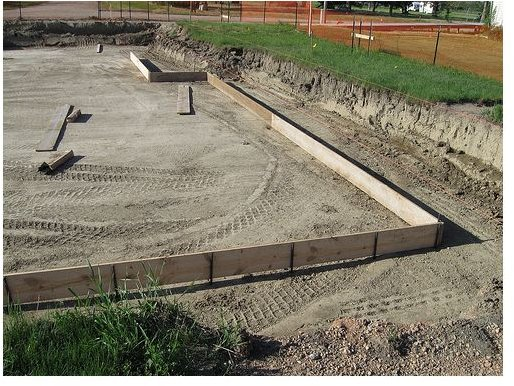# Concrete Cubic Yard Formula and Other Useful Concrete Calculations

## IntroductionRead this article to find out how to perform some useful concrete calculations. The concrete cubic yard formula is one of the most useful, and can be used by engineers, contractors, and anyone else working with concrete; it doesn’t require intense mathematics of any sort. A bags of concrete calculator is also linked, though this can easily be calculated from the provided formula. Read on further to find out how much concrete weighs.

Source: Flickr

## Concrete Cubic Yards formula

The concrete cubic yards formula is a formula of volume, and as such requires three dimensions: length, width, and depth. This formula can be used for many applications, such as pouring a foundation or a driveway. Follow the steps below to calculate the total number of cubic yards required.

• To start with, you’ll need to obtain these measurements with a measuring tape or some other device. Make sure to record all measurements in feet. Record length, width, and depth. Divide any inch measurements by 12 to get everything into feet.
• Multiply all measurements together, as: length * width * depth. This will give a result in cubic feet.
• To measure footings, add up the total length of the footings, then multiply by the width and depth. For instance, if you were pouring footings for a 10 ft. x 10 ft. shed, you would multiply the length (10 ft.) by the number of sides (4), and then multiply that result by the width of the footings, say 16 inches, which is 16/12=1.33 feet. So the area of the footings would be 10 * 4 * 1.33. Then just multiply by the depth of the footings to get the volume of concrete.
• Add up all the volumes you’ve calculated, then divide by 27 to get cubic yards. The reason for dividing by 27 is that there are 3 feet in a yard, so that in a cubic yard there are 3 * 3 * 3 = 27 cubic feet.

Cement trucks typically hold anywhere from 8 to 14 cubic yards of concrete, so use the cubic yard calculation to determine how many truckloads will be required. This foundation calculator may be useful for more complex calculations.

The reason for calculating in yards is that concrete bags are usually sold according to cubic yards. It is best to round up your calculations to the nearest half-yard, so that there is some room for error.

## Bags Concrete Calculator

To calculate the number of bags of concrete, first calculate the total yardage as above. Then, use the conversion table below to figure out how many bags to buy.

• Ninety forty-pound bags equal 1 cubic yard of concrete
• Sixty sixty-pound bags equal 1 cubic yard of concrete
• Forty-five eight-pound bags equal 1 cubic yard of concrete
• Or more generally: 1 cubic yard of concrete equals 3600 pounds of concrete.

## How much does concrete weigh?

As shown above, it takes 3600 pounds of concrete bags to make a cubic yard of concrete. However, this is not necessarily the weight of the concrete, as the bags are mixed with other things to make up concrete.

The density of concrete can vary substantially depending on the application it is to be used for. The approximate density, though, is 150 pounds/ft3, or 4050 pounds/yd3. In metric units, that’s 2400 kg/m3. Lightweight concrete weighs 1750 kg/m3. In English units, that’s about 109 lb/ft3 or 2950 lb/yd3. Make sure to look up the density of your specific blend of concrete, though, rather than relying on these figures.

## Conclusion

The above calculations are very useful to anyone pouring concrete. It is best to understand the calculations involved rather than simply relying on online calculations; this helps us realize when a number does or doesn’t make sense. If you understand how much a cubic yard of concrete really is, you may be more likely to realize you’ve made a mistake if you calculate a large cubic yardage of concrete.

## Sources

The following sources were used in writing this article:

Cubic Yards of Concrete

Density of Concrete

How to Calculate Cubic Yards